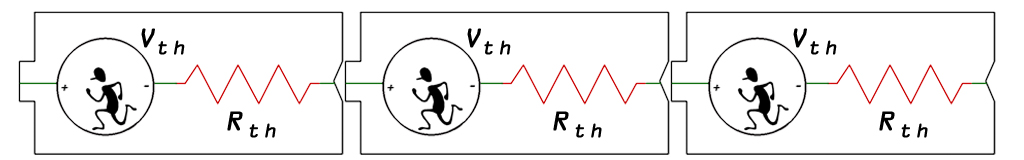# Supply Droop: Internal Resistance is Futile…What causes a battery supply voltage to drop when the load increases? Internal resistance does, and while it’s normally around 0.2 ohms per alkaline cell, here is a bit of procedure if your keen to figuring it out yourself:

• Measure open cell voltage at no load, record this as nominal voltage
• Using a known resistive load, measure the voltage under load
• Apply formula: (Nominal V – Loaded V) * (Load R) / (Nominal V)

And there you have it, an easy three step method to determine internal resistance.  For giggles I tested this bit of procedure on a new generic brand 9v battery with a 2.5 ohm power resistor as the load.  The experiment resulted in a calculated internal resistance of 1.7 ohms which is close to the expected 1.2 ohms.  A 9v battery is often assembled from 6 individual cells in series, so each cell would be about 0.28 ohms.  That’s pretty close to the 0.2 ohm per cell rule of thumb.

`Voltage Drop = Nominal Voltage - (Load Current x Internal Resistance)`

So what does this mean for your battery powered projects? In the case of high current drain applications like motors and power LEDs, one should consider the effective series resistance of the source and how it will cause the supply voltage to drop. For a known current consumption, use the above formula to figure out the drop.  Have fun…